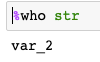Search
•Magdalena Konkiewicz

# Top 8 magic commands in jupyter notebook

Updated: Nov 13, 2020Image by Larisa Koshkina from Pixabay

What are the magic commands?

Magic commands are special commands that can help you with running and analyzing data in your notebook. They add a special functionality that is not straight forward to achieve with python code or jupyter notebook interface.

Magic commands are easy to spot within the code. They are either proceeded by % if they are on one line of code or by %% if they are written on several lines.

In this article, I am going to list magic commands that are used most often and show practical examples of how to take an advantage of the functionality they provide.

1. List all magic commands.

Let's start by listing all possible magic commands that you can use in the notebook.

`%lsmagic`

If you run the line above in your notebook you should get a list similar to the screenshot below. These are all the commands available to you. We will go through just a fraction of them in this article.2. Run a file.

You can run a python file from your jupyter notebook using the following code.

`%run <file name>`

Imagine that you have a file hello.py with the following content:

```def hello_world():
print('Hello, world')

hello_world()

```

You can run the following command in the notebook to run the file.

`%run hello.py`3. Get an execution time.

You can time the execution of the code using the time command.

```%%time

Let's generate 1 000 000 random numbers and see how long it takes.

```%%time
import random
for i in range(0, 1000000):
random.random()```4. List all variables.

There is a magic command that allows you to list all variables that are defined within the current notebook.

`%who`

You can pass it a data type after command name to list only variables of the specific data type.

To illustrate this let's define a string variable and two int variables.

```var_1 = 1
var_2 = 'hello'
var_3 = 100```

Now we can list all strings with:

`%who str`Or all integers with:

`%who int`5. Get detailed information about the variable.

Once we know a variable name we can inspect what are the details of the objects that is stored in the particular variable name. In order to do it, you can use the following command.

`%pinfo <variable>`

Let's go back to the example with three variables that we used in the previous section to illustrate it better.

```var_1 = 1
var_2 = 'hello'
var_3 = 100```

Let's now inspect var_1.

`%pinfo var_1`As you can see you get all the important information about the variable such as type, string form, and docstring.

Let's inspect a variable that is a string.

`%pinfo var_3`You get the same information details as with an int and additionally length of the string.

6. Get and set environmental variables.

You can get a list of environmental variables using the following command.

`%env`Additionally, you can also set an environmental variable with the notebook by specifying the variable name and its value.

Let’s add a new environmental variable called NEW_VAR:

`%env NEW_VAR=Users/konki`And let's now check if it has been set properly. You can add a variable name after the %env to check only a specified variable instead of displaying all of them.

`%env NEW_VAR`7. Displaying matlpotlib graphs in jupyter notebook

If you are using an older version of Jupyter notebooks you need to add this line of code so the graphs created with matplotlib can render within the notebook itself.

`%matplotlib inline`

In the newer versions, this is no longer needed. The code that sets it up is run when the Jupyter notebook is launched.

You still see this magic command a lot in other people's code as not everyone is aware of the update.

You can load an external file to a cell by using the load command.

`%load <file_name>`

This is a very useful command if you already have a python file with certain functions defined and you need to use them in the current notebook.

In order to illustrate we take an example of the file rectangle.py which consists of the following code.

```def calculate_area(len, height):
return len * height * 2```

You can load the file content by executing the code below.

`%load rectangle.py`

Once you run the cell you should get the content of the file within the cell.Now just run the cell with the loaded code and you will be able to use the functions that were defined within the original file. You can now calculate the rectangle area.

`calculate_area(2,4)`Summary

In this article, you have learned about the most used magic commands in jupyter notebooks. It's your time now, open the notebook and practice what you have learned here. Happy coding!